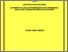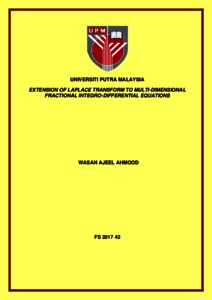# Extension of laplace transform to multi-dimensional fractional integro-differential equations

## Citation

Ahmood, Wasan Ajeel (2017) Extension of laplace transform to multi-dimensional fractional integro-differential equations. Doctoral thesis, Universiti Putra Malaysia.

## Abstract

The main focus of this thesis is to extend the study of one-dimensional fractional to multi-dimensional fractional calculus and study of multi-dimensional Laplace transformation with their respective applications. This extension will be used to solve special types of multi-dimensional fractional calculus such as space-time partial fractional derivative. The multi-dimensional Laplace transforms method used to solve the multi-dimensional fractional calculus with constant and variable coefficients and the multidimensional modification of Hes variational iteration method to solve the multi-dimensional fractional integro-differential equations with non-local boundary conditions are developed. The study of multi-dimensional space-time fractional derivative with their applications and also, new fractional derivative and integral including Riemann-Liouville having a non-local and non-singular kernel are detailed. Finally, we obtained the exact solution of multi-dimensional fractional calculus, space-time partial fractional derivative and the system of matrix fractional differential equation in Riemann- Liouville sense of matrices but there are some problems that cannot be solved analytically, thus we solved them by multi-dimensional variational iteration method. This study shows that integral transform can be used to present new solutions to problems by certain applications for solving them.Preview
Text
FS 2017 42 IR.pdfView Item